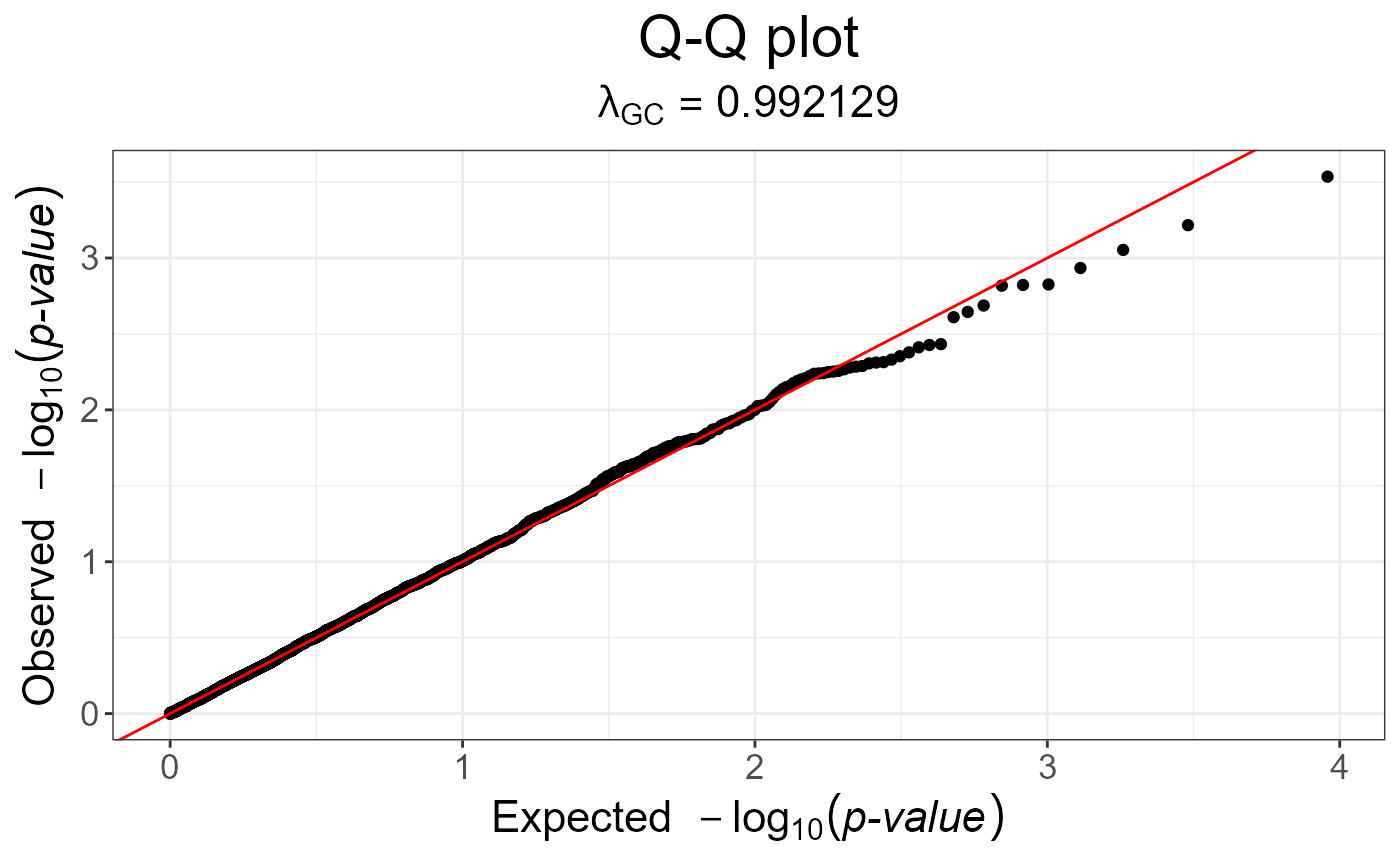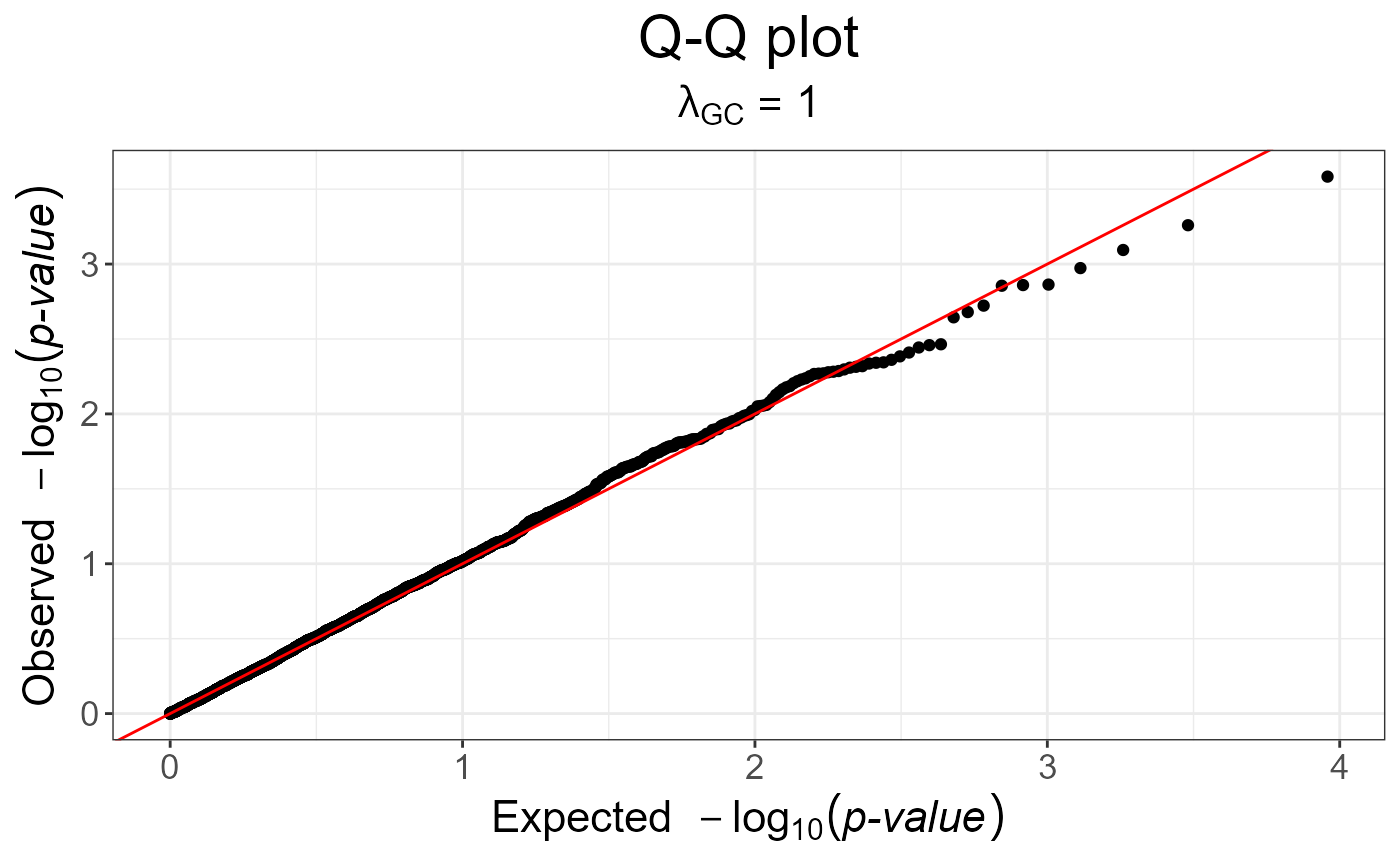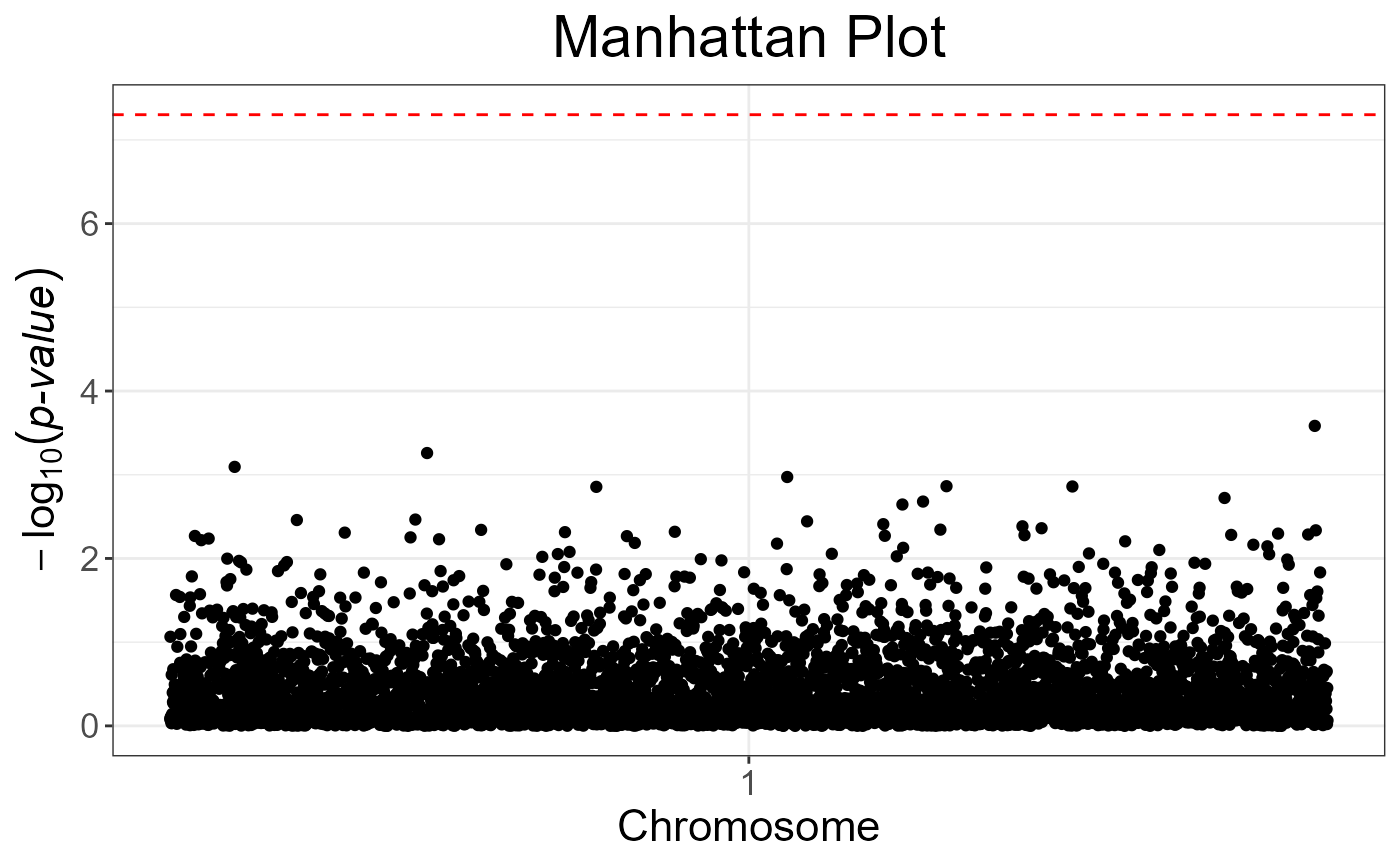Creates a manhattan plot.

snp_manhattan(
gwas,
infos.chr,
infos.pos,
colors = c("black", "grey60"),
dist.sep.chrs = 1e+07,
ind.highlight = integer(0),
col.highlight = "red",
labels = NULL,
npoints = NULL,
coeff = 1
)

## Arguments

gwas A mhtest object with the p-values associated with each SNP. Typically, the output of big_univLinReg, big_univLogReg or snp_pcadapt. Vector of integers specifying each SNP's chromosome. Typically $map$chromosome. Vector of integers specifying the physical position on a chromosome (in base pairs) of each SNP. Typically $map$physical.pos. Colors used for each chromosome (they are recycled). Default is an alternation of black and gray. "Physical" distance that separates two chromosomes. Default is 10 Mbp. Indices of SNPs you want to highlight (of interest). Default doesn't highlight any SNPs. Color used for highlighting SNPs. Default uses red. Labels of the x axis. Default uses the number of the chromosome there are in infos.chr(sort(unique(infos.chr))). This may be useful to restrict the number of labels so that they are not overlapping. Number of points to keep (ranked by p-value) in order to get a lighter object (and plot). Default doesn't cut anything. If used, the resulting object will have an attribute called subset giving the indices of the kept points. Relative size of text. Default is 1.

## Value

A ggplot2 object. You can plot it using the print method. You can modify it as you wish by adding layers. You might want to read this chapter to get more familiar with the package ggplot2.

## Details

If you don't have information of chromosome and position, you should simply use plot instead.

## Examples

set.seed(9)

test <- snp_attachExtdata()
G <- test$genotypes y <- rnorm(nrow(G)) gwas <- big_univLinReg(G, y) snp_qq(gwas)gwas_gc <- snp_gc(gwas) # change attr(gwas_gc, "transfo") snp_qq(gwas_gc)# The next plot should be prettier with a real dataset snp_manhattan(gwas_gc, infos.chr = test$map$chromosome, infos.pos = test$map$physical.pos)p <- snp_qq(gwas_gc) + ggplot2::aes(text = asPlotlyText(test$map))
if (FALSE) plotly::ggplotly(p, tooltip = "text")